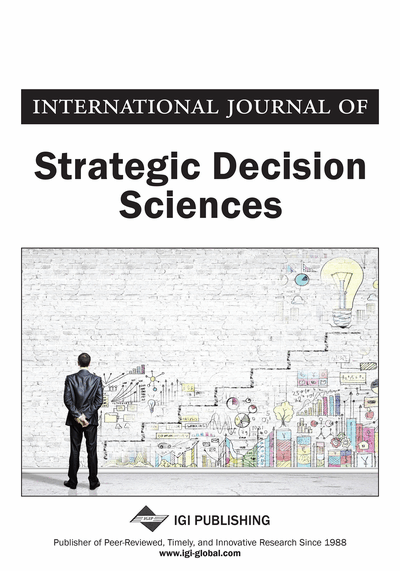# New Dual Parameter Quasi-Newton Methods for Unconstrained Nonlinear Programs

Issam A.R. Moughrabi (Gulf University for Science and Technology, Mubarak Al-Abdullah, Kuwait) and Saeed Askary (Gulf University for Science and Technology, Mubarak Al-Abdullah, Kuwait)
DOI: 10.4018/IJSDS.2019070105

## Abstract

A framework model of multi-step quasi-Newton methods developed which utilizes values of the objective function. The model is constructed using iteration genereted data from the m+1 most recent iterates/gradient evaluations. It hosts double free parameters which introduce a certain degree of flexibility. This permits the interpolating polynomials to exploit available computed function values which are otherwise discarded and left unused. Two new algorithms are derived for those function values incorporated in the update of the inverse Hessian approximation at each iteration to accelerate convergence. The idea of incorporating function values configure quasi-Newton methods, but the presentation constitutes a new approach for such algorithms. Several earlier works only include function values data in the update of the Hessian approximation numerically to improve the convergence of Secant-like methods. The methods are a useful tool for solving nonlinear problems arising in engineering, physics, machine learning, decision science, approximations techniques to Bayesian Regressors and a variety of numerical analysis applications.
Article Preview
Top

## Background

The starting-point for the development of quasi-Newton methods is the Newton equation (Broyden, 1970), which prescribes a condition which the Hessian (evaluated at a specified point) must satisfy. The “Newton Equation”, which may be regarded as a generalization of the “Secant Equation” (Byrd et al., 1988; Dennis and Schnable, 1979), is usually employed in the construction of quasi-Newton methods for optimization. According to Ortize et al. (2004): “Many designed experiments require the simultaneous optimization of multiple responses. A common approach is to use a desirability function combined with an optimization algorithm to find the most desirable settings of the controllable factors” (p. 432).

This work directs attention to problems of the form:

## Complete Article List

Search this Journal:
Reset
Open Access Articles: Forthcoming
Volume 11: 4 Issues (2020): Forthcoming, Available for Pre-Order
Volume 10: 4 Issues (2019)
Volume 9: 4 Issues (2018)
Volume 8: 4 Issues (2017)
Volume 7: 4 Issues (2016)
Volume 6: 4 Issues (2015)
Volume 5: 4 Issues (2014)
Volume 4: 4 Issues (2013)
Volume 3: 4 Issues (2012)
Volume 2: 4 Issues (2011)
Volume 1: 4 Issues (2010)
View Complete Journal Contents Listing